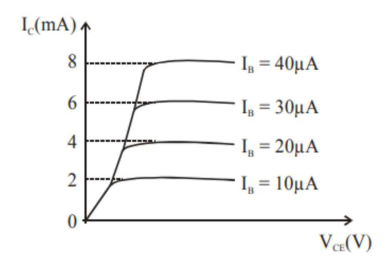# The typical output characteristics curve for a transistor working in the common-emitter configuration is shown in the figure.

Question:

The typical output characteristics curve for a transistor working in the common-emitter configuration is shown in the figure.The estimated current gain from the figure is

Solution:

(200)

$\beta=\frac{\Delta \mathrm{I}_{\mathrm{C}}}{\Delta \mathrm{I}_{\mathrm{B}}}=\frac{2 \times 10^{-3}}{10 \times 10^{-6}}$

$\beta=\frac{1}{5} \times 10^{3}$

$\beta=2 \times 10^{2}$

$\beta=200$